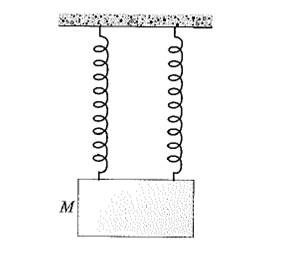# Dual SpringsA block of mass $M=3\text{ kg}$ is initially in equilibrium and hanging by 2 identical springs as shown in figure above. If one of the springs is cut from the lower point at $t=0$, then find acceleration of block in $\text{m/s}^2$ at $t=0$.

Details and Assumptions:

• Do not consider rotational effects of the block.

• Take $g=-10\text{ m/s}^2$.

• Take upward as positive direction

×

Problem Loading...

Note Loading...

Set Loading...